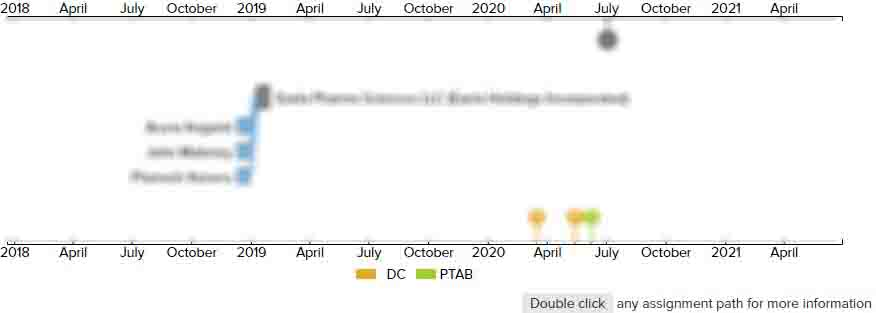# Video-encoding method and video-encoding apparatus using prediction units based on encoding units determined in accordance with a tree structure, and video-decoding method and video-decoding apparatus using prediction units based on encoding units determined in accordance with a tree structure

• US 10,412,411 B2
• Filed: 02/23/2018
• Issued: 09/10/2019
• Est. Priority Date: 04/13/2010
• Status: Active Grant
##### First Claim
Patent Images

1. A method of decoding a video, the method comprising:

• receiving a bitstream with respect to an encoded video;

extracting, from the received bitstream, coding unit structure information indicating a size of a coding unit that is a data unit for decoding of a picture of the encoded video, a variable depth of the coding unit, information about a coded depth and information about an encoding mode indicating one among prediction types including skip mode, inter mode and intra mode;

determining a current coding unit based on the coding unit structure information, the variable depth of the coding unit and the information about the coded depth;

determining a prediction type of the current coding unit based on the information about an encoding mode;

performing prediction on at least one prediction unit of the current coding unit according to the prediction type to obtain prediction samples of the current coding unit; and

determining reconstructed samples of the current coding unit by using the prediction samples,wherein the determining the current coding unit comprises;

when the information about the coded depth indicates a split for a current depth, splitting the current coding unit of the current depth into coding units of a lower depth, independently from neighboring coding units, andwherein the performing the prediction on the at least one prediction unit of the current coding unit comprises;

when the information about the coded depth indicates a non-split of a current depth, determining a prediction unit from the current coding unit;

when the prediction type is the skip mode, determining the prediction unit having a same size as the current coding unit;

when the prediction type is one of the intra mode and the inter mode, extracting partition type information indicating a partition of the current coding unit from the bitstream;

when the prediction type is the intra mode, determining the prediction unit including at least one square block, from the current coding unit, based on the partition type information; and

when the prediction type is the inter mode, determining the prediction unit including one of a partition, symmetric partitions and asymmetric partitions, from the current coding unit, based on the partition type information,wherein the symmetric partitions are obtained by symmetrically splitting at least one of a height and a width of the current coding unit,wherein the asymmetric partitions are obtained by asymmetrically splitting one of the height and the width of the current coding unit.

• ##### 0 Assignments
Timeline View
Assignment View×
×